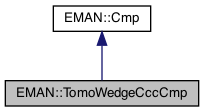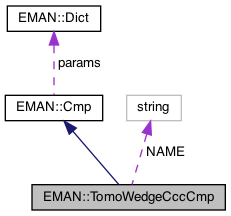EMAN2
EMAN::TomoWedgeCccCmp Class Reference

Both images should be FFTs. More...

`#include <cmp.h>`

Inheritance diagram for EMAN::TomoWedgeCccCmp:[legend]
Collaboration diagram for EMAN::TomoWedgeCccCmp:[legend]

## Public Member Functions

virtual float cmp (EMData *image, EMData *with) const
To compare 'image' with another image passed in through its parameters. More...

virtual string get_name () const
Get the Cmp's name. More...

virtual string get_desc () const

TypeDict get_param_types () const
Get Cmp parameter information in a dictionary. More...Public Member Functions inherited from EMAN::Cmp
virtual ~Cmp ()

virtual Dict get_params () const
Get the Cmp parameters in a key/value dictionary. More...

virtual void set_params (const Dict &new_params)
Set the Cmp parameters using a key/value dictionary. More...

static CmpNEW ()

## Static Public Attributes

static const string NAME = "ccc.tomo.thresh"Protected Member Functions inherited from EMAN::Cmp
void validate_input_args (const EMData *image, const EMData *with) constProtected Attributes inherited from EMAN::Cmp
Dict params

## Detailed Description

Both images should be FFTs.

Computes a CCC excluding pixels with very small value (assumes they are missing wedge) Returns overlap amount as "fft_overlap" in "image"

Date
2010-10-18

Definition at line 352 of file cmp.h.

## ◆ cmp()

 float TomoWedgeCccCmp::cmp ( EMData * image, EMData * with ) const
virtual

To compare 'image' with another image passed in through its parameters.

An optional transformation may be used to transform the 2 images.

Parameters
 image The first image to be compared. with The second image to be comppared.
Returns
The comparison result. Smaller better by default

Implements EMAN::Cmp.

Definition at line 691 of file cmp.cpp.

692{
693 ENTERFUNC;
694 EMData *imageo=image;
695 EMData *witho=with;
696 if (!image->is_complex()) image=image->do_fft();
697 if (!with ->is_complex()) with =with ->do_fft();
698 if (image->get_xsize()!=with->get_xsize() || image->get_ysize()!=with->get_ysize() || image->get_zsize()!=with->get_zsize()) throw InvalidCallException("Error: TomoWedgeCccCmp requires same sized images");
699
700 float sigmaimg = params.set_default("sigmaimg",0.5f);
701 float sigmawith = params.set_default("sigmawith",0.5f);
702 int negative = params.set_default("negative",1);
703
704 // Note 'sigma' is the STD of real and imag values treated independently
705 // s1 and s2 are threshold values squared (for speed)
706 float s1=pow((float)image->get_attr("sigma")*sigmaimg,(float)2.0);
707 float s2=pow((float)with->get_attr("sigma")*sigmawith,(float)2.0);
708
709
710 double sum=0;
711 double sumsq1=0;
712 double sumsq2=0;
713 double norm=0;
714 for (int z=0; z<image->get_zsize(); z++) {
715 for (int y=0; y<image->get_ysize(); y++) {
716 for (int x=0; x<image->get_xsize(); x+=2) {
717 float v1r=image->get_value_at(x,y,z);
718 float v1i=image->get_value_at(x+1,y,z);
719 float v1=Util::square_sum(v1r,v1i);
720 if (v1<s1) continue;
721
722 float v2r=with->get_value_at(x,y,z);
723 float v2i=with->get_value_at(x+1,y,z);
724 float v2=Util::square_sum(v2r,v2i);
725 if (v2<s2) continue;
726
727 sum+=v1r*v2r+v1i*v2i;
728 sumsq1+=v1;
729 if (Util::is_nan(sumsq1)) { printf("TomoWedgeCccCmp: NaN encountered: %d %d %d %f %f %f\n",x,y,z,v1r,v1i,v1); }
730 sumsq2+=v2;
731 norm+=1.0;
732 }
733 }
734 }
735 imageo->set_attr("fft_overlap",(float)(2.0*norm/(image->get_xsize()*image->get_ysize()*image->get_zsize())));
736// printf("%f\t%f\t%f\t%f\t%f\n",s1,s2,sumsq1,sumsq2,norm);
737
738 if (imageo!=image) delete image;
739 if (witho!=with) delete with;
740
741 if (negative) sum*=-1.0;
742 return float(sum/(sqrt(sumsq1)*sqrt(sumsq2)));
743}
Dict params
Definition: cmp.h:132
type set_default(const string &key, type val)
Default setting behavior This can be achieved using a template - d.woolford Jan 2008 (before there wa...
Definition: emobject.h:569
EMData stores an image's data and defines core image processing routines.
Definition: emdata.h:82
static bool is_nan(const float number)
tell whether a float value is a NaN
Definition: util.h:105
static float square_sum(float x, float y)
Calcuate (x*x + y*y).
Definition: util.h:764
EMData * sqrt() const
return square root of current image
bool is_complex() const
Is this a complex image?
#define InvalidCallException(desc)
Definition: exception.h:348
#define ENTERFUNC
Definition: log.h:48
#define y(i, j)
Definition: projector.cpp:1516
#define x(i)
Definition: projector.cpp:1517

## ◆ get_desc()

 virtual string EMAN::TomoWedgeCccCmp::get_desc ( ) const
inlinevirtual

Implements EMAN::Cmp.

Definition at line 362 of file cmp.h.

363 {
364 return "Ccc of two FFTs with missing wedge taken into account explicitly. Also stores overlap as 'fft_overlap' in \
365first input image. Individual voxels are include only if they exceed the threshold value in both images. fft_overlap expresses \
366the fractional number of voxels included in the comparison. Actual returned correlations already account for this effect, but \
367the overlap can be used to estimate the relative uncertainty in the resulting correlation coefficient.";
368 }

## ◆ get_name()

 virtual string EMAN::TomoWedgeCccCmp::get_name ( ) const
inlinevirtual

Get the Cmp's name.

Each Cmp is identified by a unique name.

Returns
The Cmp's name.

Implements EMAN::Cmp.

Definition at line 357 of file cmp.h.

358 {
359 return NAME;
360 }
static const string NAME
Definition: cmp.h:386

References NAME.

## ◆ get_param_types()

 TypeDict EMAN::TomoWedgeCccCmp::get_param_types ( ) const
inlinevirtual

Get Cmp parameter information in a dictionary.

Each parameter has one record in the dictionary. Each record contains its name, data-type, and description.

Returns
A dictionary containing the parameter info.

Implements EMAN::Cmp.

Definition at line 375 of file cmp.h.

376 {
377 TypeDict d;
378// d.put("norm", EMObject::BOOL,"Whether the cross correlation image should be normalized (should be for normalized images). Default is true.");
379// d.put("ccf", EMObject::EMDATA,"The ccf image, can be provided if it already exists to avoid recalculating it");
380 d.put("sigmaimg", EMObject::FLOAT, "Sigma coefficient for thresholding values included in the dot product. default = 0.5");
381 d.put("sigmawith", EMObject::FLOAT, "Sigma coefficient for thresholding values included in the dot product in the 'with' image. Default = 0.5");
382 d.put("negative", EMObject::INT, "If set, returns -1 * ccc product. Set by default so smaller is better");
383 return d;
384 }

References EMAN::EMObject::FLOAT, EMAN::EMObject::INT, and EMAN::TypeDict::put().

## ◆ NEW()

 static Cmp * EMAN::TomoWedgeCccCmp::NEW ( )
inlinestatic

Definition at line 370 of file cmp.h.

371 {
372 return new TomoWedgeCccCmp();
373 }

## ◆ NAME

 const string TomoWedgeCccCmp::NAME = "ccc.tomo.thresh"
static

Definition at line 386 of file cmp.h.

Referenced by get_name().

The documentation for this class was generated from the following files: